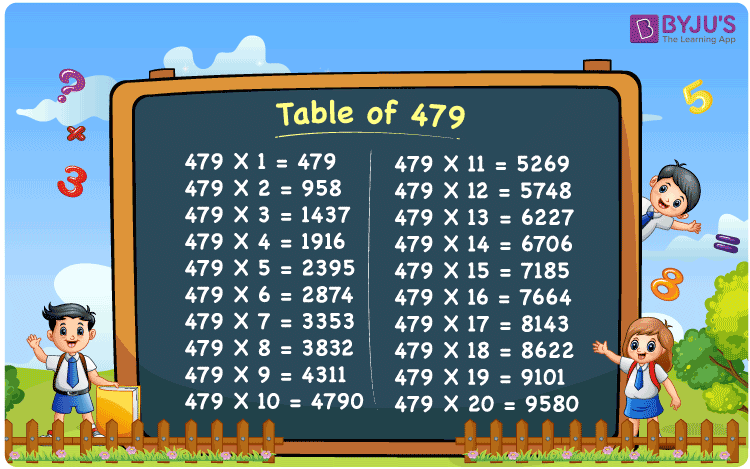Checkout JEE MAINS 2022 Question Paper Analysis : Checkout JEE MAINS 2022 Question Paper Analysis :

# Table of 479

The Table of 479 has all the required details, which is important for the students from an exam point of view. The subject matter experts prepare the multiplication tables with utmost care to help students ace the exam, irrespective of their understanding abilities. For more knowledge, students can access the multiplication table of 479 by just clicking on the download PDF link provided below. The table of 479 PDF is 100% accurate and can be trusted by the students to improve their mental calculation abilities.

## Table of 479 Chart## What is the 479 Times Table?

The multiplication table of 479 has to be memorised effectively by the students to speed up their calculations during the exams. Apart from the board exams, students also should also focus on the competitive exams to achieve their career goals. The concept of repetitive addition is discussed here in brief to help students become confident and score well in the exams.

 479 × 1 = 479 479 479 × 2 = 958 479 + 479 = 958 479 × 3 = 1437 479 + 479 + 479 = 1437 479 × 4 = 1916 479 + 479 + 479 + 479 = 1916 479 × 5 = 2395 479 + 479 + 479 + 479 + 479 = 2395 479 × 6 = 2874 479 + 479 + 479 + 479 + 479 + 479 = 2874 479 × 7 = 3353 479 + 479 + 479 + 479 + 479 + 479 + 479 = 3353 479 × 8 = 3832 479 + 479 + 479 + 479 + 479 + 479 + 479 + 479 = 3832 479 × 9 = 4311 479 + 479 + 479 + 479 + 479 + 479 + 479 + 479 + 479 = 4311 479 × 10 = 4790 479 + 479 + 479 + 479 + 479 + 479 + 479 + 479 + 479 + 479 = 4790

## Multiplication Table of 479

The Table of 479 helps students to build a strong foundation of the fundamental concepts in Mathematics. If a student is thorough with the multiplication tables right from their primary education itself, understanding the important concepts would be very easy in their higher levels. For this purpose, students can find the multiplication table of 479 till the number 20 given here in the form of a table.

 479 × 1 = 479 479 × 2 = 958 479 × 3 = 1437 479 × 4 = 1916 479 × 5 = 2395 479 × 6 = 2874 479 × 7 = 3353 479 × 8 = 3832 479 × 9 = 4311 479 × 10 = 4790 479 × 11 = 5269 479 × 12 = 5748 479 × 13 = 6227 479 × 14 = 6706 479 × 15 = 7185 479 × 16 = 7664 479 × 17 = 8143 479 × 18 = 8622 479 × 19 = 9101 479 × 20 = 9580

## Solved Example on the Table of 479

Q. At a school, 2 parents attended the function in a day. How many parents will attend the function in 479 days?

Solution: Given,

Number of parents who attended the function in a day = 2

In 479 days number of parents who will attend the function = 479 x 2

= 958

## Frequently Asked Questions on the Table of 479

### Determine the value of 479 multiplied by 12.

In the table of 479,
479 multiplied by 12 is 479 x 12 = 5748.

### Calculate 479 times 4 plus 479 times 8 minus 479 times 3.

Using the table of 479,
479 x 4 = 1916
479 x 8 = 3832
479 x 3 = 1437
So we get (479 x 4) + (479 x 8) – (479 x 3) = 1916 + 3832 – 1437
= 4311
Therefore, 479 times 4 plus 479 times 8 minus 479 times 3 is 4311.

### Find 479 times 13 plus 2 minus 6.

From the table of 479,
479 times 13 plus 2 minus 6 can be written as
479 x (13 + 2 – 6) = 479 x 11
= 5269